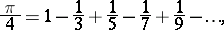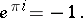# Pi(number)

(diff) ← Older revision | Latest revision (diff) | Newer revision → (diff)
Jump to: navigation, search

The ratio of the length of a circle to its diameter; it is an infinite non-periodic decimal numberOne frequently arrives at the numberas the limit of certain arithmetic sequences involving simple laws. An example is Leibniz' serieswhich, however, converges very slowly. There are more rapidly-converging series suitable for calculating.

The possibility of a pure analytic definition ofis of essential significance for geometry. For example,also participates in certain formulas in non-Euclidean geometry, but not as the ratio of the length of a circle to its diameter (this ratio is not constant in non-Euclidean geometry). The arithmetic nature ofwas finally elucidated in analysis, with a decisive part played by Euler's formula:At the end of the 18th century, J. Lambert and A. Legendre established thatis an irrational number, while in the 19th century, F. Lindemann showed thatis a transcendental number.

#### Comments

A nice account of Lindemann's proof can be found in [a3], Chapt. 6.

The number of known digits ofhas increased exponentially in recent times. At the moment (1990), the record seems to be half a billion digits (D.V. Chudnovsky and G.V. Chudnovsky). For an account of such computations see [a1]. Up to the 1960's the standard way to calculatewas to use Machin's formulaand the power series of. Nowadays, some powerful formulas of Ramanujan are used. It is still not known how randomly the digits ofare distributed; in particular, whetheris a normal number.

How to Cite This Entry:
Pi(number). Encyclopedia of Mathematics. URL: http://encyclopediaofmath.org/index.php?title=Pi(number)&oldid=11364
This article was adapted from an original article by Material from the article "Pi" in BSE-3 (originator), which appeared in Encyclopedia of Mathematics - ISBN 1402006098. See original article